# 27. Euler's Equations

Michael Fowler

## Introduction

We've just seen that by specifying the rotational direction and the angular phase of a rotating body using Euler's angles, we can write the Lagrangian in terms of those angles and their derivatives, and then derive equations of motion. These can be solved to describe precession, nutation, etc.

One might hope for a more direct Newtonian approach -- we know, for example, that the steadily precessing child's top is easy to understand in terms of the gravitational torque rotating the angular momentum vector. What about applying(the external torque on the system) more generally? It's certainly valid. The problem is that in the lab frame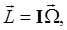meaningand the elements of the inertia tensor relative to the lab axes are constantly changing as the body rotates. The Newtonian approach is only practicable if the connection between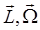can be made in the body frame defined by the principal axes of inertiain which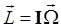is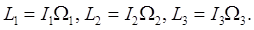With the body frame rotating at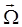relative to the fixed-in-space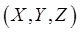frame, the rates of change of a vector in the two frames satisfy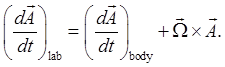To understand this equation, think first of a moving particle, say a bug crawling about on the rotating body. The bug's movement through space (meaning the lab) is equal to its movement relative to axes fixed in the rotating body, plus the rotational movement of that body relative to the fixed-in-space axes.

You might be thinking at this point: yes, I can see this is true if the vector represents the position of a particle that's moving around in space, but we're looking at the changing angular momentum, why isn't the angular momentum just zero in a frame in which the body is at rest? And the angular velocity, too? But what is meant here by the vector in the body frame is the components of the vector in an inertial frame that is momentarily coincident with the principal axes. The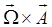term represents the change on going through this succession of inertial frames. Think of a long forward pass of a football. The ball is spinning about its long axis (usually), but that axis itself is precessing about the line of flight. Of course, air resistance presumably helps it line up this way, but is not the main effect, which is that the angular momentum vector points in a constant direction in space, the axis of symmetry is precessing around it, as we saw on throwing the ball in class. Imagine now running alongside the ball, holding a pencil pointing in the direction of the constant angular momentum vector. As seen by an observer in the ball, relative to the ball's principal axes frame of reference, the pencil will be describing a cone -- this is what we mean by the path of the angular momentum vector relative to the body axes.

The equations of motion in the body frame are thenwhere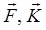are the external force and couple respectively.

Writing the angular momentum equation in components along the principal axes: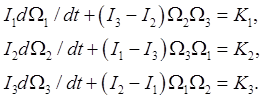These are Euler's Equations.

In the important special case of zero torque:## Free Rotation of a Symmetric Top Using Euler's Equations

This is a problem we've already solved, using Lagrangian methods and Euler angles, but it's worth seeing just how easy it is using Euler's equations.

For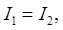the third equation gives immediately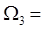constant.

Then, writing for convenience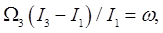the first two equations areThese equations can be combined to give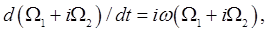soThat is,moves around a circle centered at the origin with constant angular velocity. So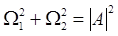stays constant, and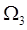is constant, so the total angular velocity vector has constant length and rotates steadily about the body-frame axisFrom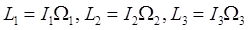, and rememberit follows that the angular momentum vector also precesses at a steady rate aboutin the body frame. (We know that in the fixed space frame, the angular momentum vector is constant!)

It follows that, as viewed in the external (lab) frame, the fixed-in-the-body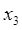axis (and therefore the body) precesses around the constant angular momentum vector at a steady rate.

Of course, the rate is the same as that found using Euler's angles, recall from the previous lecture thatso in precessionand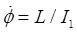so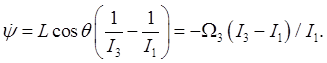## Using Energy and Angular Momentum Conservation

We can also gain some insight into the motion of the free spinning top just from conservation of energy and angular momentum.

The equations are: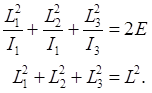Visualize these equations as surfaces in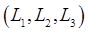space.

The second is a sphere, radius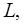centered at the origin.

The first is an ellipsoid, also centered at the origin, with semimajor axes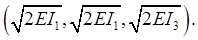Do these two surfaces intersect? The answer is yes, they always do. Assuming first that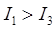, then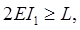and further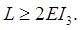The sphere intersects the ellipsoid in two circles. These degenerate to one circle, the equator, when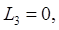and two points (the poles) when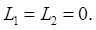We conclude that the path of the angular momentum vector is a circle around the axis of symmetry in the body coordinate system, and since we know that relative to the fixed space axes the angular momentum is in fact constant, this means that actually the body is precessing about its axis of symmetry as seen by an observer.

## The Asymmetrical Top

Here we'll generalize the above equations and argument to the general case of three different moments of inertia about the three principal axes. That is,We'll take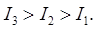From the limitations on energy for given angular momentum, the maximum sphere radius is the maximum semimajor axis of the ellipsoid, the ellipsoid touches the sphere at its two extreme poles. For a slightly smaller sphere, the lines of intersection arte two small ellipses centered at the poles, evidently the major axis will go around this elliptical cone in fixed space. At the other extreme, the minimum allowed angular momentum for a given energy, the sphere is entirely inside the ellipsoid except that it touches at the ends of the shortest axes. Again, for a top with slightly more angular momentum, it will precess (elliptically) around this minimum axis.

In both these cases, spin about the axis is stable against small perturbations. This is not the case for spin about the middle axis -- for that energy, the intersection of the sphere and ellipsoid does not reduce to two points.

The equations for pure spin about the middle axis can be written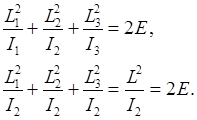These equations define the points common to the sphere and the ellipsoid at this energy.Subtracting the second from the first, we find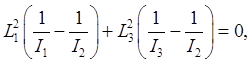with solutions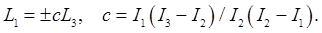So for this energy and total angular momentum squared, the intersection of the sphere and ellipsoid is in two planes, both containing the intermediate axis. This means that any perturbation of this motion will send the system along one of these paths, or a trajectory close to it -- in other words, it will deviate far from its original motion, in contrast to spin about either of the other two axes.

In all cases, the path followed by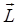, the intersection of the sphere and the ellipsoid, is closed, so the angular momentum cycles back to its original value, in periodic motion. Landau calculates the period, a straightforward (but too lengthy to repeat here) solution of Euler's equations, giving elliptic functions. Actually, the top does not return to its original configuration: the angular momentum returns, but the top has a different angular position about its axis. Landau states this result, but refers to Whittaker for the derivation.